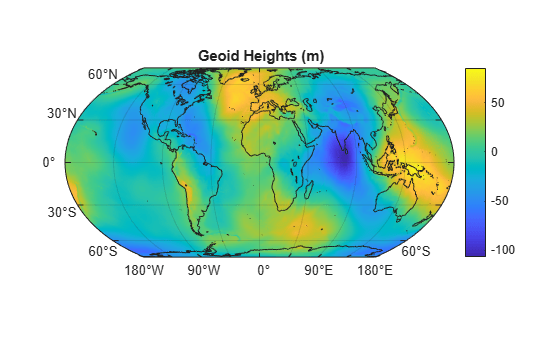# egm96geoid

Geoid height from Earth Gravitational Model 1996 (EGM96)

Syntaxes of the `egm96geoid` function that return referencing vectors will be removed in a future release. Use a syntax that returns a reference object instead (requires R2020a or later). For more information, see Compatibility Considerations.

## Syntax

``N = egm96geoid(lat,lon)``
``N = egm96geoid(R)``
``[N,globalR] = egm96geoid``

## Description

example

````N = egm96geoid(lat,lon)` returns the height in meters of the geoid at the specified latitude and longitude from the Earth Gravitational Model of 1996 (EGM96). Specify latitude and longitude in degrees. (since R2019b)```

example

````N = egm96geoid(R)` returns geoid heights at the cell center or posting locations specified by the geographic postings reference or geographic cells reference object `R`. (since R2020a)```

example

````[N,globalR] = egm96geoid` returns geoid heights for the entire globe as a 721-by-1441 matrix spaced at 15-minute intervals with latitude limits `[-90 90]` and longitude limits `[0 360]`. The function also returns a geographic postings reference object that contains spatial referencing information for the geoid heights. (since R2020a)```

## Examples

collapse all

Find geoid heights from EGM96 by specifying latitude and longitude values in degrees. The result is returned in meters.

```lat = 27.988056; lon = 86.925278; N = egm96geoid(lat,lon)```
```N = -28.7444 ```

View geoid heights from EGM96 for an area including Europe.

First, create a `GeographicPostingsReference` object. Specify the latitude and longitude limits of the area in degrees. Specify the raster size as the number of rows and columns for the grid of geoid heights. Then, return the grid of geoid heights for the area by calling `egm96geoid` on the reference object.

```latlim = [35 72]; lonlim = [-12 51]; rasterSize = [100 100]; R = georefpostings(latlim,lonlim,rasterSize); N = egm96geoid(R);```

Note that the size of `N` matches the raster size of `R`.

`size(N)`
```ans = 1×2 100 100 ```

Load coastline latitude and longitude data into the workspace. Then, plot the geoid height and coastline data on a set of map axes.

```load coastlines worldmap(latlim,lonlim) geoshow(N,R,'DisplayType','surface') geoshow(coastlat,coastlon,'Color','k')```View geoid heights from EGM96 for the entire globe.

First, return the geoid heights and a referencing object for the globe. The geoid heights are spaced at 15-minute intervals. Load coastline latitude and longitude data into the workspace. Then, plot the geoid heights and coastline data on a set of map axes.

```[N,R] = egm96geoid; load coastlines worldmap('World') geoshow(N,R,'DisplayType','surface') geoshow(coastlat,coastlon,'Color','k')```## Input Arguments

collapse all

Latitude in degrees, specified as a scalar, vector, or matrix. The dimension of `lat` depends on the dimension of the geoid heights you want to find.

• To find a single geoid height, specify `lat` and `lon` as scalars.

• To find several geoid heights, specify `lat` and `lon` as vectors of the same length.

• To find a p-by-q grid of geoid heights, specify `lat` and `lon` as p-by-q matrices.

Data Types: `single` | `double`

Longitude in degrees, specified as a scalar, vector, or matrix. The dimension of `lon` depends on the dimension of the geoid heights you want to find.

• To find a single geoid height, specify `lat` and `lon` as scalars.

• To find several geoid heights, specify `lat` and `lon` as vectors of the same length.

• To find a p-by-q grid of geoid heights, specify `lat` and `lon` as p-by-q matrices.

Data Types: `single` | `double`

Geographic reference, specified as a `GeographicPostingsReference` object or a `GeographicCellsReference` object that contains geospatial referencing information for `N`. The `RasterSize` property of the geographic reference object determines the size of the data grid, `size(N)`.

## Output Arguments

collapse all

Geoid height in meters, returned as a scalar, vector, or matrix.

The size of `N` depends on the syntax:

SyntaxSize of `N`
`N = egm96geoid(lat,lon)`Size of `lat` and `lon`
`N = egm96geoid(R)``R.RasterSize`
`[N,globalR] = egm96geoid`721-by-1441 matrix

Data Types: `double`

Geographic reference, returned as a `GeographicPostingsReference` object of size 721-by-1441 with latitude limits `[-90 90]` and longitude limits `[0 360]`.

collapse all

### Earth Gravitational Model of 1996 (EGM96)

The geoid is an equipotential surface of the Earth's gravity field that approximates global mean sea level. You can visualize the geoid as the surface of the ocean without effects such as weather, waves, and land. The Earth Gravitational Model of 1996 (EGM96) is based on the ellipsoid specified by the World Geodetic System of 1984 (WGS84), so the `egm96geoid` function returns heights above or below the surface of the WGS84 ellipsoid.

## Version History

Introduced before R2006a

expand all

Warns starting in R2022a